Author: Oscar Cronquist Article last updated on January 30, 2019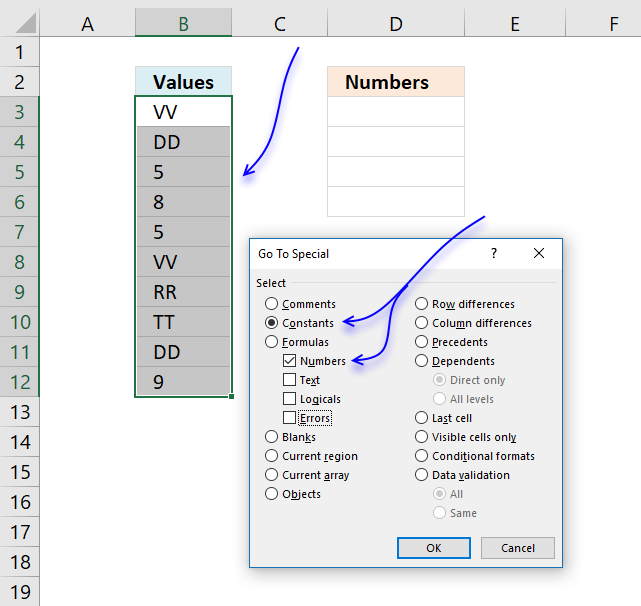I this article I will show you how to get numerical values from a cell range manually and using an array formula. Lets get started, the steps below demonstrates how to select only numbers from cell range B3:B12 manually.

You don't need to select all cells containing numbers one by one, this trick willl save you a lot of time.

1. Select cell range B3:B12 with your mouse.
2. Press function key F5 on your keyboard.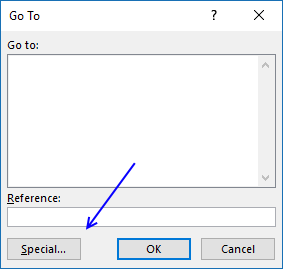3. A dialog box appears, click "Special..." button.
4. Another dialog box shows up, click "Constants".
Note, if your selection contains formula then click on "Formulas".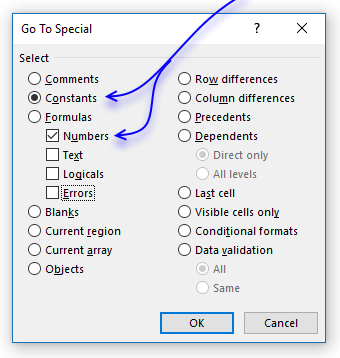5. Make sure checkbox "Numbers" is selected only, see image above.
6. Click OK button.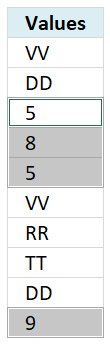7. The selection changes to only selecting numbers, see image above.
8. Copy these cells. (CTRL + c)
9. Select a destination cell with your mouse.
10. Paste selection. (Ctrl + v)

The image below demonstrates an array formula that extracts numbers only, this can be useful in a dashboard or interactive worksheets.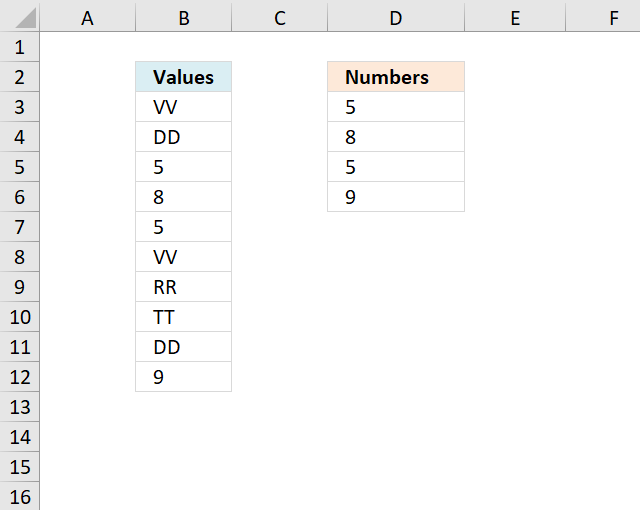Question: I want to extract all numeric values into a new column?

If you have both letters and digits in a cell then read this article:
How to extract numbers from a cell value

Array formula in C2:

=INDEX(\$B\$3:\$B\$12, SMALL(IF(ISNUMBER(\$B\$3:\$B\$12), ROW(\$B\$3:\$B\$12)-MIN(ROW(\$B\$3:\$B\$12))+1, ""), ROWS(D2:\$D\$2)))

To enter an array formula, type the formula in a cell then press and hold CTRL + SHIFT simultaneously, now press Enter once. Release all keys.

The formula bar now shows the formula with a beginning and ending curly bracket telling you that you entered the formula successfully. Don't enter the curly brackets yourself.

### Explaining formula in cell D3

#### Step 1 - Check if a value is a number

The ISNUMBER function returns TRUE if value is a number.

ISNUMBER(\$B\$3:\$B\$12)

becomes

ISNUMBER({"VV"; "DD"; 5; 8; 5; "VV"; "RR"; "TT"; "DD"; 9})

and returns

{FALSE; FALSE; TRUE; TRUE; TRUE; FALSE; FALSE; FALSE; FALSE; TRUE}

#### Step 2 - Convert TRUE to corresponding row number

The IF function returns corresponding row number if the logical test is TRUE and nothing "" if the logical test is FALSE.

IF(ISNUMBER(\$B\$3:\$B\$12), ROW(\$B\$3:\$B\$12)-MIN(ROW(\$B\$3:\$B\$12))+1, "")

becomes

IF({FALSE; FALSE; TRUE; TRUE; TRUE; FALSE; FALSE; FALSE; FALSE; TRUE}, ROW(\$B\$3:\$B\$12)-MIN(ROW(\$B\$3:\$B\$12))+1, "")

becomes

IF({FALSE; FALSE; TRUE; TRUE; TRUE; FALSE; FALSE; FALSE; FALSE; TRUE}, {1;2;3;4;5;6;7;8;9;10}, "")

and returns

{""; ""; 3; 4; 5; ""; ""; ""; ""; 10}

#### Step 3 - Return k-th smallest row number

The SMALL function makes sure that a new value is returned in each cell.

SMALL(IF(ISNUMBER(\$B\$3:\$B\$12), ROW(\$B\$3:\$B\$12)-MIN(ROW(\$B\$3:\$B\$12))+1, ""), ROWS(D2:\$D\$2))

becomes

SMALL({""; ""; 3; 4; 5; ""; ""; ""; ""; 10}, ROWS(D2:\$D\$2))

The ROWS function returns the number of rows in a cell reference, in this case, the cell reference expands when the cell is copied to cells below.

SMALL({""; ""; 3; 4; 5; ""; ""; ""; ""; 10}, ROWS(D2:\$D\$2))

becomes

SMALL({""; ""; 3; 4; 5; ""; ""; ""; ""; 10}, 1)

and returns 3.

#### Step 4 - Return value

The INDEX function returns a value based on a row and column number.

INDEX(\$B\$3:\$B\$12, SMALL(IF(ISNUMBER(\$B\$3:\$B\$12), ROW(\$B\$3:\$B\$12)-MIN(ROW(\$B\$3:\$B\$12))+1, ""), ROWS(D2:\$D\$2)))

becomes

INDEX(\$B\$3:\$B\$12, 3)

and returns 5 in cell D3.

### Download Excel fileList-all-numbers-in-a-column.xlsx